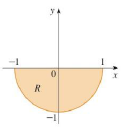Chapter 15.3, Problem 3E

Chapter
Section
Textbook Problem

A region R is shown. Decide whether to use polar coordinates or rectangular coordinates and write ∬ R f ( x ,   y )   d A as an iterated integral, where f is an arbitrary continuous function on R.3.To determine

To write: An iterated integral by deciding whether to use polar coordinates or rectangular coordinates.

Explanation

Formula used:

If f is a polar rectangle R given by 0arb,αθβ, where 0βα2π , then, Rf(x,y)dA=αβabf(rcosθ,rsinθ)rdrdθ (1)

Calculation:

Since the given region is the semicircle, it is easier to use polar coordinates.

From the figure given in the problem, it is observed that radius r ranges from 0 to 1 and θ varies from π to 2π .

Therefore, the polar coordinates is, R={(r,θ)|0r1,πθ2π}

Still sussing out bartleby?

Check out a sample textbook solution.

See a sample solution

The Solution to Your Study Problems

Bartleby provides explanations to thousands of textbook problems written by our experts, many with advanced degrees!

Get Started

Find more solutions based on key concepts